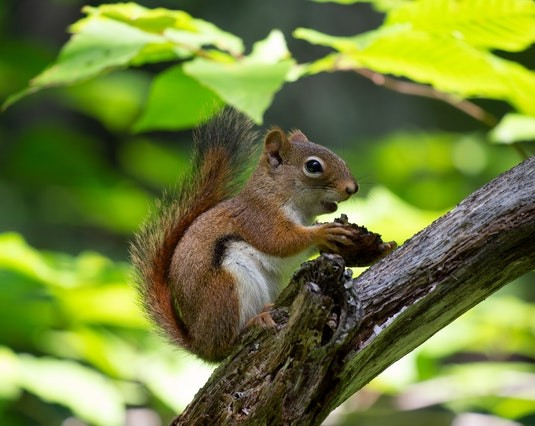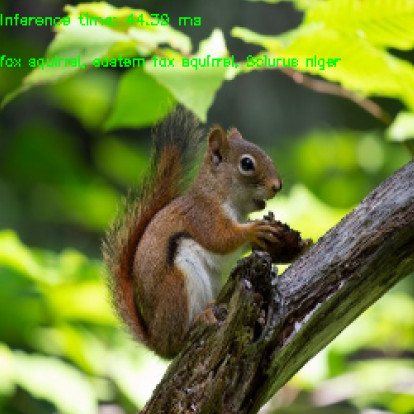OpenCV  4.8.0-dev Open Source Computer Vision
Conversion of PyTorch Classification Models and Launch with OpenCV C++
Original author Anastasia Murzova
Compatibility OpenCV >= 4.5

## Goals

In this tutorial you will learn how to:

• convert PyTorch classification models into ONNX format
• run converted PyTorch model with OpenCV C/C++ API
• provide model inference

We will explore the above-listed points by the example of ResNet-50 architecture.

## Introduction

Let's briefly view the key concepts involved in the pipeline of PyTorch models transition with OpenCV API. The initial step in conversion of PyTorch models into cv::dnn::Net is model transferring into ONNX format. ONNX aims at the interchangeability of the neural networks between various frameworks. There is a built-in function in PyTorch for ONNX conversion: torch.onnx.export. Further the obtained .onnx model is passed into cv::dnn::readNetFromONNX or cv::dnn::readNet.

## Requirements

To be able to experiment with the below code you will need to install a set of libraries. We will use a virtual environment with python3.7+ for this:

virtualenv -p /usr/bin/python3.7 <env_dir_path>
source <env_dir_path>/bin/activate

For OpenCV-Python building from source, follow the corresponding instructions from the Introduction to OpenCV.

Before you start the installation of the libraries, you can customize the requirements.txt, excluding or including (for example, opencv-python) some dependencies. The below line initiates requirements installation into the previously activated virtual environment:

pip install -r requirements.txt

## Practice

In this part we are going to cover the following points:

1. create a classification model conversion pipeline
2. provide the inference, process prediction results

### Model Conversion Pipeline

The code in this subchapter is located in the samples/dnn/dnn_model_runner module and can be executed with the line:

python -m dnn_model_runner.dnn_conversion.pytorch.classification.py_to_py_resnet50_onnx

The following code contains the description of the below-listed steps:

1. instantiate PyTorch model
2. convert PyTorch model into .onnx
# initialize PyTorch ResNet-50 model
original_model = models.resnet50(pretrained=True)
# get the path to the converted into ONNX PyTorch model
full_model_path = get_pytorch_onnx_model(original_model)
print("PyTorch ResNet-50 model was successfully converted: ", full_model_path)

get_pytorch_onnx_model(original_model) function is based on torch.onnx.export(...) call:

# define the directory for further converted model save
onnx_model_path = "models"
# define the name of further converted model
onnx_model_name = "resnet50.onnx"
# create directory for further converted model
os.makedirs(onnx_model_path, exist_ok=True)
# get full path to the converted model
full_model_path = os.path.join(onnx_model_path, onnx_model_name)
# generate model input
generated_input = Variable(
torch.randn(1, 3, 224, 224)
)
# model export into ONNX format
torch.onnx.export(
original_model,
generated_input,
full_model_path,
verbose=True,
input_names=["input"],
output_names=["output"],
opset_version=11
)

After the successful execution of the above code we will get the following output:

PyTorch ResNet-50 model was successfully converted: models/resnet50.onnx

The proposed in dnn/samples module dnn_model_runner allows us to reproduce the above conversion steps for the following PyTorch classification models:

• alexnet
• vgg11
• vgg13
• vgg16
• vgg19
• resnet18
• resnet34
• resnet50
• resnet101
• resnet152
• squeezenet1_0
• squeezenet1_1
• resnext50_32x4d
• resnext101_32x8d
• wide_resnet50_2
• wide_resnet101_2

To obtain the converted model, the following line should be executed:

python -m dnn_model_runner.dnn_conversion.pytorch.classification.py_to_py_cls --model_name <pytorch_cls_model_name> --evaluate False

For the ResNet-50 case the below line should be run:

python -m dnn_model_runner.dnn_conversion.pytorch.classification.py_to_py_cls --model_name resnet50 --evaluate False

The default root directory for the converted model storage is defined in module CommonConfig:

@dataclass
class CommonConfig:
output_data_root_dir: str = "dnn_model_runner/dnn_conversion"

Thus, the converted ResNet-50 will be saved in dnn_model_runner/dnn_conversion/models.

### Inference Pipeline

Now we can use models/resnet50.onnx for the inference pipeline using OpenCV C/C++ API. The implemented pipeline can be found in samples/dnn/classification.cpp. After the build of samples (BUILD_EXAMPLES flag value should be ON), the appropriate example_dnn_classification executable file will be provided.

To provide model inference we will use the below squirrel photo (under CC0 license) corresponding to ImageNet class ID 335:

fox squirrel, eastern fox squirrel, Sciurus nigerClassification model input image

For the label decoding of the obtained prediction, we also need imagenet_classes.txt file, which contains the full list of the ImageNet classes.

In this tutorial we will run the inference process for the converted PyTorch ResNet-50 model from the build (samples/build) directory:

./dnn/example_dnn_classification --model=../dnn/models/resnet50.onnx --input=../data/squirrel_cls.jpg --width=224 --height=224 --rgb=true --scale="0.003921569" --mean="123.675 116.28 103.53" --std="0.229 0.224 0.225" --crop=true --initial_width=256 --initial_height=256 --classes=../data/dnn/classification_classes_ILSVRC2012.txt

Let's explore classification.cpp key points step by step:

Net net = readNet(model, config, framework);

The model parameter value is taken from --model key. In our case, it is resnet50.onnx.

• preprocess input image:
if (rszWidth != 0 && rszHeight != 0)
{
resize(frame, frame, Size(rszWidth, rszHeight));
}
// Create a 4D blob from a frame
blobFromImage(frame, blob, scale, Size(inpWidth, inpHeight), mean, swapRB, crop);
// Check std values.
if (std.val != 0.0 && std.val != 0.0 && std.val != 0.0)
{
// Divide blob by std.
divide(blob, std, blob);
}

In this step we use cv::dnn::blobFromImage function to prepare model input. We set Size(rszWidth, rszHeight) with --initial_width=256 --initial_height=256 for the initial image resize as it's described in PyTorch ResNet inference pipeline.

It should be noted that firstly in cv::dnn::blobFromImage mean value is subtracted and only then pixel values are multiplied by scale. Thus, we use --mean="123.675 116.28 103.53", which is equivalent to [0.485, 0.456, 0.406] multiplied by 255.0 to reproduce the original image preprocessing order for PyTorch classification models:

img /= 255.0
img -= [0.485, 0.456, 0.406]
img /= [0.229, 0.224, 0.225]
• make forward pass:
net.setInput(blob);
Mat prob = net.forward();
• process the prediction:
Point classIdPoint;
double confidence;
minMaxLoc(prob.reshape(1, 1), 0, &confidence, 0, &classIdPoint);
int classId = classIdPoint.x;

Here we choose the most likely object class. The classId result for our case is 335 - fox squirrel, eastern fox squirrel, Sciurus niger:ResNet50 OpenCV C++ inference output